# CS202 -- Lab 5

• CS202 -- Data Structures
• James S. Plank
• Fall, 2021
• Directory for files, makefile, executables and gradescript: /home/jplank/cs202/Labs/Lab5
• This is a two week lab.

## To begin

Do the following:
```UNIX> cp -r /home/jplank/cs202/Labs/Lab5/src .
UNIX> cp -r /home/jplank/cs202/Labs/Lab5/include .
UNIX> cp -r /home/jplank/cs202/Labs/Lab5/files .
UNIX> cp /home/jplank/cs202/Labs/Lab5/makefile .
UNIX> mkdir obj
UNIX> mkdir bin
```

## You are not allowed...

You are not allowed to modify include/bitmatrix.hpp or src/bitmatrix_editor.cpp.

## Bit-matrices

This lab is all about bit-matrices. These are matrices whose values can only be zero or one, and arithmetic is modulo 2. You would be surprised how powerful these are. They are used in fault-tolerant storage applications, among other things, which is why I am fond of them.

Let's take some simple examples. Here are four bit-matrices:

 files/C003.txt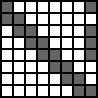files/C244.txt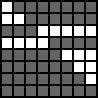files/RV.txt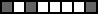files/CV.txt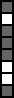When you add bit-matrices, you simply add elements in corresponding rows and columns, modulo two. So, for example:+=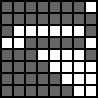and+=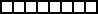Bit-matrix multiplication is just like standard matrix multiplication, except that addition and multiplication of the individual elements is done modulo two. For example, multiplying files/RV.txt and files/CV.txt will yield a 1 X 1 matrix whose value is:

(1*1) + (0*0) + (1*1) + (0*1) + (0*1) + (0*0) + (0*0) + (1*1)

This is 1+0+1+0+0+0+0+1 which equals 3%2 which equals 1. Thus:*=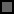Similarly:*=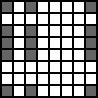*=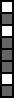and*=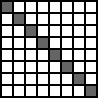That last example is interesting -- when the product of two square matrices is equal to the identity matrix, then these two matrices are inverses of each other. Some matrices, like the one below, have no inverses:### Bitmatrix.hpp

You have a big job -- to implement the Bitmatrix class, plus the BM_Hash class and four additional procedures that work with bitmatrices. Pretty much all explanation is in the header file, include/bitmatrix.hpp:

 ```#pragma once #include #include class Bitmatrix { public: /* Bitmatrix creation constructors / methods. */ Bitmatrix(int rows, int cols); /* Create an empty bitmatrix with the given size. Throw the string "Bad rows" if (rows <= 0). Throw the string "Bad cols" if (cols <= 0). */ Bitmatrix(const std::string &fn); /* Read the bitmatrix from a file. Throw "Can't open file" if you can't open the file. Throw "Bad file format" if you can't read the file. */ Bitmatrix *Copy() const; /* Create a new bitmatrix using new, which is a copy of the caller's bitmatrix, and return a pointer to it. */ /* Bitmatrix storage methods. */ bool Write(const std::string &fn) const; /* Write to a file. You will print one line per row of the bitmatrix, and each line is only composed of 0's and 1's. Return true if successful and false if not. */ void Print(size_t w) const; /* Print on standard output. You will print one line per row of the bitmatrix. Each line is composed of 0's and 1's, and there will be a space after every w characters (don't put a space at the end if the number of columns is a multiple of w). Also put a blank line after every w rows (except the last, if the number of rows is a multiple of w). */ bool PGM(const std::string &fn, /* Create a PGM file. Each entry is a p by p square, */ int p, /* which is white for zero and 100 for gray. If border is */ int border) const; /* greater than zero, then there should be a black border of that many pixels separating each square and around the whole matrix. Return true if successful and false if not. */ /* Bitmatrix access methods. */ int Rows() const; /* Return the number of rows */ int Cols() const; /* Return the number of columns */ char Val(int row, int col) const; /* Return the specified element ('0' or '1'). Return 'x' if row or col is bad. */ /* Bitmatrix modification methods. */ bool Set(int row, int col, char val); /* Set the specified element to val. Val must be 0, 1, '0' or '1'. If val is 0 or 1, store '0'/'1' in the matrix. Return true if successful and false if not. */ bool Swap_Rows(int r1, int r2); /* Swap these rows. Return true if successful and false if not. */ bool R1_Plus_Equals_R2(int r1, int r2); /* Set the row r1 to the sum of row r1 and r2. Return true if successful and false if not. */ protected: std::vector M; /* The matrix. Elements are '0' or '1'. */ }; /* These four procedures will allocate and create a new bitmatrix from other bitmatrices. They must be written using the methods of the Bitmatrix class above. They should return NULL if they are unsuccessful. */ Bitmatrix *Sum(const Bitmatrix *a1, const Bitmatrix *a2); Bitmatrix *Product(const Bitmatrix *a1, const Bitmatrix *a2); Bitmatrix *Sub_Matrix(const Bitmatrix *a1, const std::vector &rows); Bitmatrix *Inverse(const Bitmatrix *m); /* We are also going to support storage and retrieval of bitmatrices through a hash table. */ class HTE { /* This is a "hash table entry". Our hash table stores */ public: /* vectors of pointers to these, using separate chaining. */ std::string key; Bitmatrix *bm; }; class BM_Hash { /* This is our bitmatrix hash table. */ public: BM_Hash(int size); /* You specify the table size in the constructor. Throw the string "Bad size" if (size <= 0). */ bool Store(const std::string &key, Bitmatrix *bm); /* Store a bitmatrix with the given key. Return true if successful and false if not. Return false if the key is already there. */ Bitmatrix *Recall(const std::string &key) const; /* Retrieve a bitmatrix with the given key. Return NULL if unsuccessful. */ std::vector All() const; /* Return all of the hash table entries. */ protected: std::vector < std::vector > Table; /* This is the hash table. */ }; ```

• Bitmatrix(string fn) reads bitmatrix files in a specific format:
• Blank lines are ignored.
• Non-blank lines must be composed solely of zeros, ones or whitespace.
• Each non-blank line represents a row of the matrix (with whitespace ignored).
• Each row has to have the same number of columns.

• void Print(int w): Print the matrix on standard output. If w is less than or equal to zero, print each row on its own line with no spaces. Otherwise, print a space after every w columns and a blank line after every w rows.

• void PGM(string fn, int pixels, int border): Create a PGM file from the bit-matrix. This is how I made the pictures above. The zero entries are white (255) and the one entries are gray (100). Each entry is a pixels by pixels square. If border is greater than zero, then there should be a black (0) border of that many pixels separating each square and around the whole matrix. The above matrices were created with PGM(xxx, 10, 2).

The only piece of data in a bitmatrix is a vector of strings named M. If M is storing a r by c matrix, then it will contain r strings, each of which has c characters. The characters are either '0' for zero or '1' for one.

So, you have to implement 12 methods, and only one of them (PGM()) is difficult. Were I you, I'd wait until the end to do PGM().

You have to implement four procedures which operate on pointers. Since these are not part of the data structure, you have to use Rows() Cols(), Set(), and Val() to implement them. You should not modify the input matrices in any way (the "const" keywords won't let you):

• Bitmatrix *Sum(const Bitmatrix *a1, const Bitmatrix *a2): This creates a new bit-matrix which is the sum of a1 and a2. If a1 and a2 are not the same size, return NULL.

• Bitmatrix *Product(const Bitmatrix *a1, const Bitmatrix *a2): This creates a new bit-matrix which is the product of a1 and a2. This product will have a1->Rows() rows and a2->Cols() columns. If a1->Cols() and a2->Rows() do not match, then return NULL.

• Bitmatrix *Sub_Matrix(const Bitmatrix *a1, const vector &rows): This creates a new bit-matrix composed of the specified rows of the given bit-matrix. It is ok to repeat entries in rows. However, if rows is empty or contains bad indices, return NULL.

• Bitmatrix *Inverse(const Bitmatrix *a1): Create and return the inverse of a1. To do this, you should also use the Swap_Rows() and R1_Plus_Equals_R2() methods. I'll go into more detail on how to invert a bit-matrix below. If a1 is not square or not invertible, return NULL.
The first three of these are very easy. Inverse() will be tougher.

Finally, you are also going to implement a hash table to store bit-matrices with keys that are strings. You should use the djb_hash() function from class as the hash function and you should use separate chaining as the collision resolution mechanism. Your hash table is a vector of vectors of HTE's (hash table entries). Each hash table entry stores a key and a pointer to a bit-matrix.

The BM_Hash class has four methods, which are described in the header file. A little more detail on All(): Return a vector of all hash table entries in the table. The vector should be ordered just like the hash table. In other words, suppose "A" hashes to 5, "B" hashes to 1 and "C" hashes to 1. And suppose that "B" was added to the table before "C". Then All() should return the HTE's in the order "B", "C", "A". You should not call new or delete on bit-matrices when you implement any of the hash table methods.

### Starter Code

The file src/bitmatrix_start.cpp provides dummy implementations for everything in the lab. The nice thing about it is that it will compile with any program that uses bitmatrix.hpp. However, it won't run correctly. It's a good starting place for you, so the first thing you should do is copy it to src/bitmatrix.cpp, and then start working on implementing methods.

### The testing program matrix_editor.cpp

I've written bitmatrix_editor.cpp as a program that uses all of the features of bitmatrix.hpp. You run it with an optional prompt as a command line argument. If you don't specify a prompt, it will not print a prompt. This is nice because you can treat the editor as an interactive editor, or you can write scripts for it.

Bitmatrix_editor reads lines of text from standard input. Blank lines and lines that begin with '#' are ignored. Otherwise, the first word on a line is a command and the remaining words are arguments.

At all times, there is one "current matrix." You may also store and recall matrices with single-word keys. Three simple commands are:

• READ filename: This reads a bit-matrix from a given file using the Read() method. If there is an error, it will return a single-element matrix whose element is zero.

• void Print(int w): Print the matrix on standard output. If w is equal to zero, print each row on its own line with no spaces. Otherwise, print a space after every w columns and a blank line after every w rows.

• QUIT: Exits. You can also simply close standard input with CNTL-D.
```UNIX> bin/bitmatrix_editor "BM-Editor>"
BM-Editor> PRINT
10000001
11000000
01100001
00110001
00011001
00001100
00000110
00000011
BM-Editor> PRINT 4
1000 0001
1100 0000
0110 0001
0011 0001

0001 1001
0000 1100
0000 0110
0000 0011
BM-Editor> PRINT
10100001
BM-Editor> PRINT 4
1010 0001
BM-Editor> QUIT
UNIX>
```
bitmatrix_editor also implements the following commands, which of course will only work when you implement the proper methods and procedures:
• EMPTY rows cols: Creates a bit-matrix of the given size whose values are all zeros. It sets the current matrix to this matrix.

• SET row col value: Sets the given element of the current matrix to the given value.

• VAL row col: Returns the value of the given element.

• SWAP r1 r2: Swaps the given rows of the current matrix using Swap_Rows().

• += r1 r2: Sets r1 of the current matrix equal to r1+r2 using the R1_Plus_Equals_R2() method.

• WRITE fn: Calls the Write() method to write the current matrix to the specified file.

• PGM fn pixels border: Calls the PGM() method to create a PGM picture of the current matrix.

• STORE key: Makes a copy of the current matrix and stores it into the hash table with the given key. If the key is already in the hash table, it should return false.

• RECALL key: If the key is in the hash table, this will set the current matrix to a copy of the bit-matrix stored in the hash table.

• ALL key: This calls All() and prints out each key, along with the dimensions of the bit-matrix stored there.

• SUM key1 key2: This adds the two matrices stored with the given keys and puts the sum into the current matrix.

• PRODUCT key1 key2: This multiplies the two matrices stored with the given keys and puts the product into the current matrix.

• SUB row1 row2 ...: This creates a submatrix of the current matrix with the specified rows, and replaces the current matrix with the submatrix.

• INVERT: This inverts the current matrix and replaces it with the inverse.

Some examples with the above matrices:

```UNIX> bin/bitmatrix_editor 'BM-Editor>'
BM-Editor> STORE C003
BM-Editor> STORE C244
BM-Editor> STORE CV
BM-Editor> STORE RV
BM-Editor> STORE t3
BM-Editor> STORE t4
BM-Editor> RECALL RV
BM-Editor> PRINT
10100001
BM-Editor> ALL
CV                               8 X   1
RV                               1 X   8
t3                              16 X   8
t4                               8 X  16
C003                             8 X   8
C244                             8 X   8
BM-Editor> SUM C003 C003
BM-Editor> PRINT
00000000
00000000
00000000
00000000
00000000
00000000
00000000
00000000
BM-Editor> SUM C003 C244
BM-Editor> PRINT
11111110
11111111
10000001
00111110
11100001
11110000
11111000
11111100
BM-Editor> PRODUCT RV CV
BM-Editor> PRINT
1
BM-Editor> PRODUCT C003 C244
BM-Editor> PRINT
10000000
01000000
00100000
00010000
00001000
00000100
00000010
00000001
BM-Editor> PRODUCT C003 CV
BM-Editor> PRINT
0
1
0
1
1
1
0
1
BM-Editor> RECALL t3
BM-Editor> PRINT 8
10000001
11000000
01100001
00110001
00011001
00001100
00000110
00000011

01111111
00111111
11100000
00001111
11111000
11111100
11111110
11111111
BM-Editor> RECALL t4
BM-Editor> PRINT 8
10000001 01111111
11000000 00111111
01100001 11100000
00110001 00001111
00011001 11111000
00001100 11111100
00000110 11111110
00000011 11111111
BM-Editor> PRODUCT t3 t4
BM-Editor> PRINT 8
10000010 10000000
01000001 01000000
10100010 00100000
01010011 00010000
00101011 00001000
00010101 00000100
00001010 00000010
00000101 00000001

10000000 11010101
01000000 11101010
00100000 10100000
00010000 00000101
00001000 01010111
00000100 10101011
00000010 01010101
00000001 10101010
BM-Editor> PRODUCT t4 t3
BM-Editor> PRINT 8
01010111
10101011
00000010
01010110
01111100
10111110
01011111
10101111
BM-Editor> RECALL C003
BM-Editor> INVERT
BM-Editor> PRINT
01111111
00111111
11100000
00001111
11111000
11111100
11111110
11111111
BM-Editor> RECALL C244
BM-Editor> INVERT
BM-Editor> PRINT
10000001
11000000
01100001
00110001
00011001
00001100
00000110
00000011
BM-Editor> PRODUCT CV RV
BM-Editor> PRINT
10100001
00000000
10100001
10100001
10100001
00000000
00000000
10100001
BM-Editor> INVERT
Matrix not invertible.
BM-Editor> QUIT
UNIX>
```

### Inverting a bit-matrix

Inverting a bit-matrix is easier than doing a general matrix inversion. However, the steps are the same. Suppose you want to invert the square matrix M. What you do is make a copy of M, and create an identity matrix of the same size as M. Call this matrix Inv. Then you perform "SWAP" and "+=" operations on the copy of M to turn it into an identity matrix. You perform the exact same operations on Inv. When you're done, the inverse of the original matrix is in Inv

Let's perform an example on the matrix in files/Inv-Ex.txt:

 ```10110 01011 10111 11001 01100 ```

You first go through each row of M from the first to the last, doing the following steps:

• Suppose you are at row i. If M[i][i] is not one, then find a row j where j > i such that M[j][i] equals one, and swap rows i and j. If you can't find such a row, the matrix is not invertible.

• Now, look at every row j such that j > i. If M[j][i] equals one, then set row j equal to the sum of rows i and j.
We'll do this for our example:

 Action M Inv Start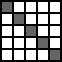i=0 No swap necesary. Set row 2 = row 2 + row 0.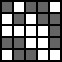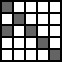i=0 still. Set row 3 = row 3 + row 0.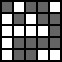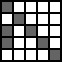i=1 No swap necesary. Set row 3 = row 3 + row 1.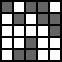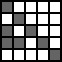i=1 still. Set row 4 = row 4 + row 1.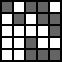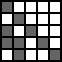i=2 Swap row 2 and row 3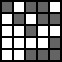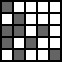i=2 still. Set row 4 = row 4 + row 2.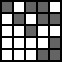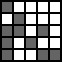i=3 Swap row 3 and row 4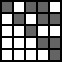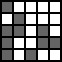When you're done with this pass, M will be an upper-triangular matrix. Now, you start with the last row and go to the first row. Suppose you are in row i:
• If there is any j > i where M[i][j] equals one, replace row i with the sum of row i and row j.
When you are done with this step, M will be the identity matrix, and Inv will be the inverse of the original M:

 Action M Inv Start: i=4 No action necessary.i=3 Set row 3 = row 3 + row 4.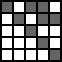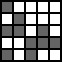i=2 No action necessary.i=1 Set row 1 = row 1 + row 3.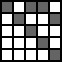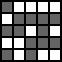i=1 still. Set row 1 = row 1 + row 4.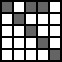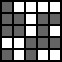i=0 Set row 0 = row 0 + row 2.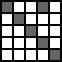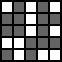i=0 still. Set row 0 = row 0 + row 3.Finally, let's double-check ourselves:

```BM-Editor> READ files/Inv-Ex.txt
BM-Editor> STORE Inv-Ex
BM-Editor> INVERT
BM-Editor> PRINT
01101
11011
11010
00111
10100
BM-Editor> STORE Inv
BM-Editor> PRODUCT Inv Inv-Ex
BM-Editor> PRINT
10000
01000
00100
00010
00001
BM-Editor>
```